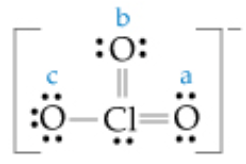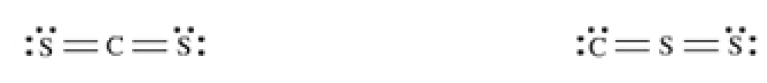# Problem: Calculate the formal charge on each of the oxygen (O) atoms labeled a, b, and c in the following Lewis structure.Express your answers as integers separated by commas.For certain molecules such as CS2, there is more than one isomer possible. For each isomer, a Lewis structure can be drawn and the formal charge on each atom can be calculated. You can use the formal charges to determine the most stable structure for a molecule. As a rule, the Lewis structure with the lowest formal charge on each atom and which places the negative charge on the most electronegative atom is generally the most stable structure.The formal charge on an atom is calculated using the following formula:formal charge = (number of valence e-) - [(number of non-bonding e-) + (12 × number of bonding e-)]CS2 as shown in the interactive activity has two isomers. In one structure carbon is the central atom, and in the other sulfur is the central atom. In the first structure, the formal charges on the S, C, and S atoms are 0, 0, and 0, respectively. In the second structure, the formal charges on the C, S, and S atoms are − 2, +2, and 0 respectively. In the second structure, it is not possible to create a resonance structure that has formal charges of zero on each atom. It is unlikely that sulfur is the central atom in this molecule.

###### FREE Expert Solution
96% (357 ratings)View Complete Written Solution
###### Problem Details

Calculate the formal charge on each of the oxygen (O) atoms labeled a, b, and c in the following Lewis structure.For certain molecules such as CS2, there is more than one isomer possible. For each isomer, a Lewis structure can be drawn and the formal charge on each atom can be calculated. You can use the formal charges to determine the most stable structure for a molecule. As a rule, the Lewis structure with the lowest formal charge on each atom and which places the negative charge on the most electronegative atom is generally the most stable structure.The formal charge on an atom is calculated using the following formula:

CS2 as shown in the interactive activity has two isomers. In one structure carbon is the central atom, and in the other sulfur is the central atom. In the first structure, the formal charges on the S, C, and S atoms are 0, 0, and 0, respectively. In the second structure, the formal charges on the C, S, and S atoms are − 2, +2, and 0 respectively. In the second structure, it is not possible to create a resonance structure that has formal charges of zero on each atom. It is unlikely that sulfur is the central atom in this molecule.